# Area Of Composite Shapes

in hive-122481 •  19 days ago

Hi there. In this post, I talk about the area of composite shapes. It is assumed that the reader is knows what perimeter is and knows how to compute perimeter of squares, rectangles, triangles and circles.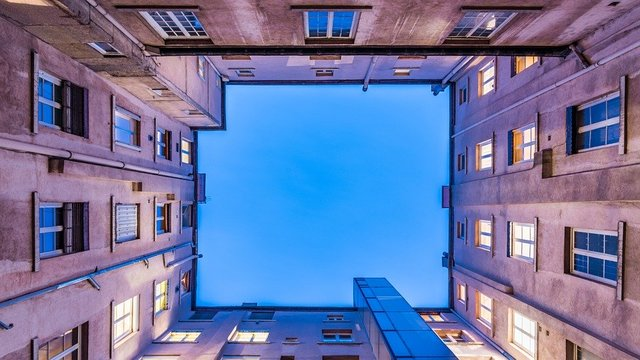Pixabay Image Source

## Topics

• What Are Composite Shapes?
• Area Of Squares & Rectangles Review
• Area Of Triangles Review
• Area Of Circles Review
• Examples Of Composite Shapes Areas

## What Are Composite Shapes?

Composite shapes is just a more fancy way of saying shapes that consist of smaller well known shapes. One example is a hockey rink being a composite shape as it consists of a rectangle with a semi circle at each end. In a basketball court there is the rectangle and semi circle composite shape for free throws.

## Area Of Squares & Rectangles Review

Computing the area of a rectangle or square is quite easy. Just simply multiply the length by the width together.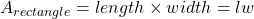The square is a special case of a rectangle where the length and width are the same. This adjusted area formula would be"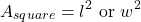Pixabay Image Source

## Area Of Triangles Review

A triangle is half of its respective parallelogram or rectangle. With this in mind the area of the triangle has a 2 in its denominator. The numerator consists of the triangle's base and the triangle's height.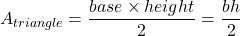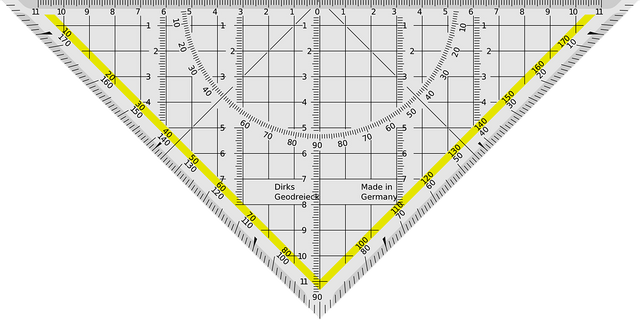Pixabay Image Source

## Area Of Circles Review

For the area of a circle, you need the circle's radius. The radius is the distance from the centre of the circle to any point on the circle's boundary. (To decimal places pi is 3.14)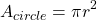Pixabay Image Source

## Examples Of Composite Shapes Areas

This section looks at finding areas of composite shapes. You will notice that a common strategy is break down the composite shape into more recognizable and manageable shapes.

Example One - L Shape

A backyard has a shape like the letter L. It looks like the picture below with its dimensions in metres.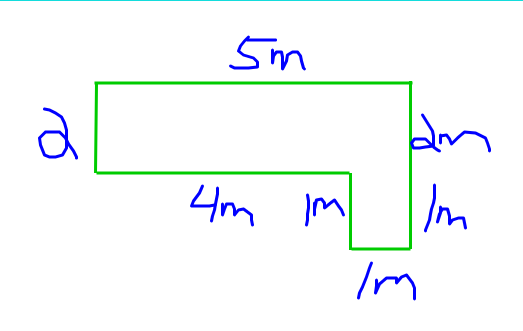It is not possible to find the area of this L Shape backyard right away. You can split this backyard into more easier shapes, compute the area of each shape and add the areas together.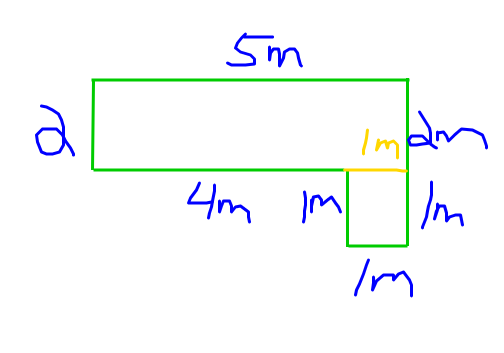The area of the larger rectangle is the product of 5 metres and 2 metres which is 10 metres. With the smaller piece, the area is 1 square metre. In total, the area of the L shaped backyard is 11 square metres.

Example Two - Ice Rink

In the picture below, an ice rink has a rectangle and two semi-circles. The radius for each semi circle is 5 metres (half of 10 metres).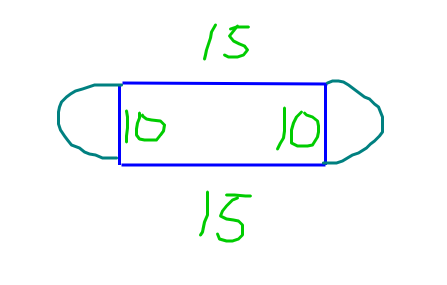The area of the ice rink is the area of the rectangle plus the area of the two semi circles. Taking the area of the two semi circles is the same as taking the area of one full circle with the same radius of 5 metres.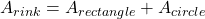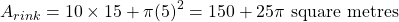This is about 228.54 square metres.

Example Three - Basketball Free Throw Area

For this example, refer to the college basketball court dimensions picture from the interwebs.

The focus is on finding the area of this section.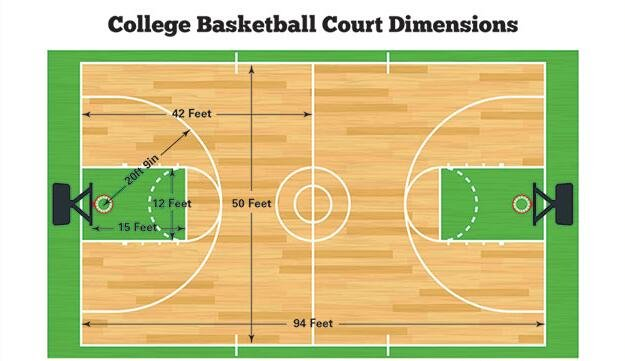Computing the area of the rectangle part is not too hard. It is 15 feet multiplied by 12 feet which is 180 square feet. For the semi-circle we do not have the radius of the semi-circle. Taking half of the 12 feet diameter gives the radius of 6 feet.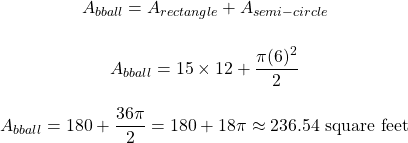Pixabay Image Source